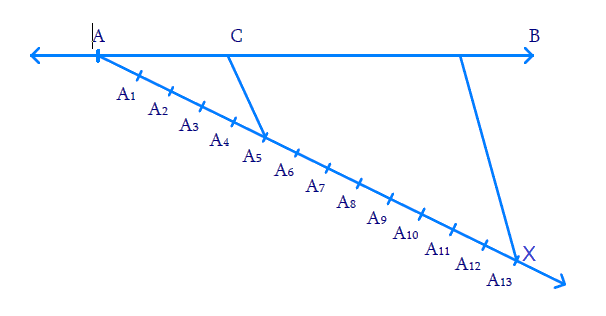# Ex.11.1 Q1 Constructions Solution - NCERT Maths Class 10

Go back to  'Ex.11.1'

## Question

Draw a line segment of length $$7.6 \,\rm{cm}$$ and divide it in the ratio $$5:8$$. Measure the two parts.

## Text Solution

#### What is known?

Length of line segment and the ratio to be divided.

Construction

#### Reasoning:

• Draw the line segment of given length.
• Then draw another line which makes an acute angle with the given line.
• Divide the line into $$m + n$$ parts where $$m$$ and $$n$$ are the ratio given.
• Basic proportionality theorem states that, “If a straight line is drawn parallel to a side of a triangle, then it divides the other two sides proportionally".

#### Steps:(i) Draw $${AB = 7.6\, \rm{cm}}$$

(ii) Draw ray $$AX,$$ making an acute angle width $$AB.$$

(iii) Mark $$13\;(=\;5\;+\;8)$$ points $${A_1},\,{A_2},\,\ldots.\,{A_13}$$ on $$AX$$ such that$$\text{A}{{\text{A}}_{\text{1}}}\text{=}\,{{\text{A}}_{\text{1}}}{{\text{A}}_{\text{2}}}\text{=}{{\text{A}}_{2}}{{\text{A}}_{3}}\text{=}.....{{\text{A}}_{\text{12}}}{{\text{A}}_{\text{13}}}$$

(iv)  Join $${{BA}_{13}}$$

(v) Through $$A_5$$ (since we need $$5$$ parts to $$8$$ parts) draw  $${{C}}{{{A}}_{{5}}}$$ parallel to $${{B}}{{{A}}_{{{13}}}}$$ where $$C$$ lies on $$AB.$$

Now $${{AC: CB = 5:8}}$$

We find $$AC = 2.9 \,\rm{cm}$$ and $$CB = 4.7 \,\rm{cm}$$

Proof:

$${{C}}{{{A}}_{{5}}}$$ is parallel to $${{B}}{{{A}}_{{{13}}}}$$

By Basic Proportionality theorem, in $${{\Delta A}}{{{A}}_{{{13}}}}{{B}}$$

\begin{align}\frac{{AC}}{{CB}} = \frac{{{{A}}{{{A}}_{{5}}}}}{{{{{A}}_{{5}}}{{{A}}_{{{13}}}}}}{{ = }}\frac{{{5}}}{{{8}}}\,\,\end{align} (By Construction)

Thus, $$C$$ divides $$AB$$ in the ratio $$5:8$$.

Learn from the best math teachers and top your exams

• Live one on one classroom and doubt clearing
• Practice worksheets in and after class for conceptual clarity
• Personalized curriculum to keep up with school